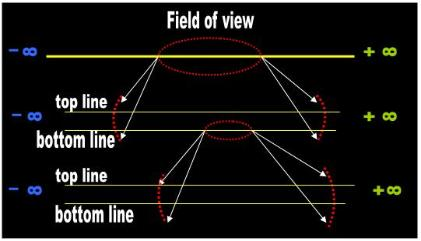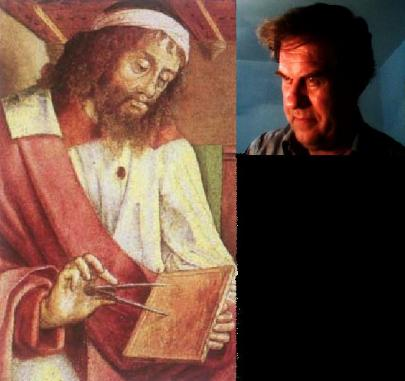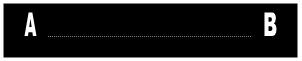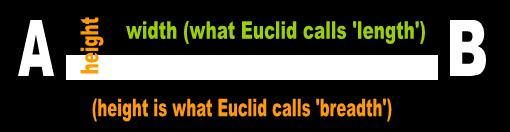There! Isn’t that better! As you can see, the ends A and B forming a greater than 0º angle with your line of
sight. In other words, you cannot visualize the first dimension from a lateral perspective because the ends
and edges form a greater than 0º angle. If you can imagine it, if you can visualize it, if you can draw it, it is
not one-dimensional! To put it in mathematical terms so that the morons of Mathematics may also
understand, in Physics, the set of geometric figures that are one-dimensional is empty! Dr. Math and others
always suspected that this was true, but they self-servingly and unjustifiably dismissed it as a trivial matter:

“Mathematicians say that their lines have zero thickness, which is pretty hard to
imagine. When we draw lines on a paper, they always have a little bit of width.
But when we study lines in geometry, we think of them as having no width at
all.” (p. 8)  

[Hard to Imagine? A little bit of width? This is a black and white issue, buddy!
Either a line  is 2-D or it is 1-D. There is no intermediate version possible. How
do you 'think' of a figure that has 'no width at all' anyways?]

What the mathematicians are trying to get away with is another of their 'convenient' and 'useful' dualities.
They want you to 'see' a standalone figure and 'assume' that it is 1-D. In this subtle manner they get the
best of both worlds. On the one hand they, point to Physics and, on the other, they describe with Math.
The mathematicians obtain these results not by carefully juggling numbers and equations, but by fiddling
around with the language. The mathematical theorist doesn't lose any sleep over 0-D points and 1-D lines
because he knows that in his abstract discipline he is not required to produce the physical object during
his presentation. Mathematical Physics is a discipline that does not and cannot follow the scientific method.
It fails at the most fundamental of step: the presentation of valid exhibits. [Read this article  if you wish to get
a more detailed analysis of my argument.]

The mathematicians want it both ways. They want you to believe that a line is a two-dimensional geometric
figure that looks like an elongated rectangle. They specifically clarify that this line extends forever. This is
what they point to and what you see. However, since they cannot use this contraption in Mathematics, the
mathematicians forget about it for the remainder of the dissertation. From now on and throughout the
explanation of the theory, a line will perhaps be a finite segment or maybe a series of numbers or the
itinerary of an apple. The mathematicians never use the contraption they pointed to at the beginning of
their presentation. This again is an unacceptable violation of the scientific method.

4.0   A dynamic geometric figure?

A more fundamental problem with Euclid's endless 'breadthless length' is that it is an itinerary and not a
geometric figure. The mathematicians still define Euclid's line in terms of 'paths':

“ A set of numerous points that extend endlessly in two directions. A line is the quickest
way to get from one point to another.”  

[Numerous points or the quickest way? Is there no difference between walking and
a row of apples?]

Straight lines, defined as the shortest paths between two neighboring points... When
a string is stretched between two points on the surface of a sphere the string is
constrained to remain in a two-dimensional space defined by the curved surface of
the sphere, in other words it has to follow the shape of the globe.” (p. 71)   

[The shortest path or a string? Is there no difference between a telephone wire and
riding on a bus?]

In non-Euclidean geometry it is more common to talk about geodesics than (straight)
lines. A geodesic is the path that a particle follows if no force is applied to it.” 

Now perhaps we can understand why Euclid was saying that his line was infinite and had no breadth. He
is not talking about a standalone figure he can point to. He is alluding to perpetual motion. For the purposes
of Physics, the word breadth is one of the three vectors (i.e., displacement). What's this got to do with the
static, structural discipline we call Geometry? Euclid's endless breadth is not a photograph, but a sculpture
still in the making. Until he is finished sculpting it, we can perhaps refer to it as the inconclusive line.

Actually, whenever a mathematician uses the words 'infinite' or 'infinity' he is alluding to something else:

Infinity can simply be beyond the limit of our understanding. 

The mathematician is saying that he doesn't have any clue of what he is talking about. In Mathematical
Physics, the word infinite is a euphemism for 'I don't know.'

5.0   Conclusions

In 3000 years, the mathematicians have not understood the true nature of their beloved Geometry, but
more significantly they do not use their strategic terms consistently. They point to a rectangle and say that
it is one-dimensional. They tell you that their figure extends infinitely, when in fact they are alluding to
perpetual motion. They point to the dimension we know as width and call it length or breadth or a path or
whatever. Or they point to a photograph and incongruously explain that you are staring at a movie. To the
Neanderthals of Mathematics it's all the same. Then, of course, it is not surprising that the lamebrains tell
you that their terms are undefined, that they invent ridiculous 'disciplines' such as String Theory, and that
should be removed from Physics and from Science. It does not qualify as a standalone geometric figure.
 Adapted for the Internet from:Why God Doesn't Exist

1.0   Euclid's perpetual line

Euclid defined a line as ‘breadthless length’ (Bk. I, Def. 2).    His disciples still use his proposal in their
dissertations:

“ In Discrete Geometry, a point is a dot. Lines are composed of an infinite set of dots in a
row.”  

“ line: A straight set of points that extends into infinity in both directions.”  

“ 1D space consists of a single straight line stretching off to infinity at either end” 

Euclid's ‘endless breadth’ (infinite line) fails for three reasons:
it is not a standalone geometric figure
it doesn't have any dimensions
it is constructed dynamically

2.0   A geometric figure?

An infinite line extends forever and, thus, you are statutorily barred from visualizing its endpoints. In effect,
Euclid deprives you of the benefit of imagining the shape of his 'figure'. To see the incomprehensible entity
that Euclid has decreed, let’s magnify his line under our Scanning Electron Microscope SEM (Fig. 1).
 Fig. 1   The bottom lineEuclid’s ‘endless breadth’(and endless parallel lines)under the SEM.

At first glance, this line segment appears to satisfy Euclid's requirements and of what we typically define
as the 1-D. Ideally, the line has a single dimension, length, and exhibits no angles greater than 0º. An
object that embodies a 45º angle, on the other hand, as a minimum has length and height (i.e., is at least
two-dimensional).

However, the reason you can see this line (although just barely) is, again, that it has two dimensions:
width and height. If we so much as imagine a line or a point, we cannot be visualizing the first of the
dimensions. We are at least in the second:

“ Stranger. But the very fact that a Line is visible implies that it possesses yet another
Dimension… If a Line were mere length without ‘height,’ it would cease to occupy Space
and would become invisible.” (Sec. 16)  

Let’s magnify Euclid’s ‘breadth-less’ length to make this absolutely clear (Fig. 3):
 Fig. 3   Absolutely breathless!Euclid’s ‘breadth-less length’ under the SEM again.I would show you what a line is, but my compass is not long enough. Do you understand the problem we mathematicians run into, Bill?Yes, Euclid, I think I do.

Upon closer inspection Euclid´s famous line consists of two parallel lines that extend beyond our field
of view and which he defines as having no ends. Objectively, what we have before us are two edges:
one at the top and another at the bottom. Conceptually, an edge is the end of a geometric figure.
Therefore, from a strictly objective,conceptual point of view, what we are staring at is not a line, but
rather two unspecified, straight-edge geometric figures that are in close proximity. This is what Euclid
defined in effect by extending his lines forever.

But let us assume that we are actually staring at a line. We must then necessarily make another
assumption: that we have not yet finished visualizing the entire object because we are standing too
close. We are not far enough to be looking at this geometric figure from a bird's-eye perspective. Okay.
No problem. However, if we magnify each of these parallel lines, lo and behold, we find that each of them
also consists of two parallel lines without endpoints. And each of these lines in turn is also comprised of
two parallel lines, and so on ad infinitum (Fig. 1) Euclid’s line is forever under construction in all four
cardinal directions. What he described is a movie of the edges of two geometric figures extending forever
in the east-west direction or of two lines comprised of two lines comprised of two lines extending in
perpetuum in the north south direction. I guess that we will just have to wait until Euclid resuscitates to
know what he meant by the word 'line'.

Meanwhile, don’t let Euclid persuade you that the magnified lines are really the edges of a continuous
geometric figure extending from top to bottom (i.e., from the edge to the south edge).

What geometric figure, Euclid? All we see is two parallel edges. Prove to us that you placed a wooden
board under the microscope! A board is surrounded by space all around, and in that case it could
conceivably qualify as a line. We have no idea what caps Euclid's alleged 'line' or whether it is capped at
all. He left it to your discretion.

3.0   Only length?

Euclid may accuse me of distorting his noble intentions and insist that his original line is a 1-D figure.  I
simply have to get used to the idea that this figure is unique in that it does not have height:

lines are intrinsically one-dimensional objects”  

“ A line, or straight line, can be described as an (infinitely) thin, (infinitely) long,
perfectly straight curve (the term curve in mathematics includes ‘straight curves’).”  

This proposal merely requires that we keep an open mind and get used to the fact that there are special
objects in Geometry.

If Euclid's line is 1-D, why is it that we can see his line? Euclid points to a standalone, 2-D board having
width and height and asks you to believe that you are staring at a 1-D edge. An edge is not a standalone
figure, but rather the boundary of an unspecified object or figure. If we allow Euclid to treat his
contraption as both a 2-D rectangle (a line) and as a 1-D edge, he can explain anything with them and
make his theories eternally  un-falsifiable. He is not using his definition consistently or, more accurately,
it amounts to a retroactive amendment of his initial assumptions.

Euclid's 1-D proposal also leads to insurmountable communications and conceptualization problems.
For instance, if a line has a single dimension, which of the three known dimensions is it?

Euclid and his successors answer: length.

First, Linear space, which has only one dimension - Length.”  

“ The 1D world is like a piece of string. There is only one axis along which one may move
along this string: the X-axis. The only dimension any object can have is length, because
there aren't any other dimensions to accommodate width or height. So the only possible
objects in 1D are points, which are 0D, and lines, which are 1D.” 

He specifically clarifies that his line is devoid of breadth and, consistent with his intentions, we will help
him out and also make it free of height. Let’s attempt to illustrate what Euclid and his Greeks are talking
 Fig. 2   Typical representation of the 1D________________________________________________________________________________________